# enzyems Flashcards

Set Details Share
created 7 years ago by Jennifer_Morales
1,748 views
exam 3 includes this chapter
updated 7 years ago by Jennifer_Morales
Subjects:
biochemistry
Page to share:
Embed this setcancel
COPY
code changes based on your size selection
Size:
X

1

the fundamental conditions for life

- ability to self-replicate

- ability to catalyzed chemicals reactions efficiently and selectively

2

What are enzymes

they are mostly proteins

some are nucleotides , catalytic RNA or Ribozymes

3

How do enzymes differ from chemical catalysts

- higher reaction rate

- milder reaction conditions

- greater specificity

- capacity for regulation

4

what do enzymes require for activity

intact protein structure

some may require cofactors, coenzymes, covalent prosthetic groups

5

what are the six reaction types of enzymes

oxidoreductases

transferases

hydrolases

lyases

isomerases

ligases

6

how do enzyme work

the presence of an active site where a substrate binds

7

how does the energy change with an enzyme

lower energy pathway from substrate to product

same overall free energy change for the reaction

8

what is need to measure free energy change?

(biochemsitry)

stander conditions

T: 298K, P:1atm , Concertation:1M

Biochemistry

pH 7 and H+ concentration is below 1MI

9

If free energy is negative

If P is lower in energy then Sspontaneous reaction

the free energy is negative and P is favored during equilibrium

10

what is the difference between the energy level between the ground state and the transition state called?

activation energy

High activation energy means slower reaction

lower activation energy means faster reaction

11

Rate limiting step

the step that has the highest activation energy

12

how do you increase reaction rates

increase temperature or increase pressure

13

how do you lower the activation energy

adding a Catalyst , they accelerate the reaction rate

14

One of the enzymes involved in glycolysis, aldolase, requires Zn2+ for catalysis. Under conditions of zinc deficiency, when the enzyme may lack zinc, it would be referred to as the:

apoenzyme

15

Which one of the following is not among the six internationally accepted classes of enzymes?

Polymerases

16

Enzymes are potent catalysts because they:

lower the activation energy for the reactions they catalyze.

17

The role of an enzyme in an enzyme-catalyzed reaction is to:

increase the rate at which substrate is converted into product.

18

Which one of the following statements is true of enzyme catalysts?

They can increase the reaction rate for a given reaction by a thousand fold or more

19

which one of the following statements is true of enzyme catalysts?

They lower the activation energy for the conversion of substrate to product.

20

Which of the following statements is false?

For S → P, a catalyst shifts the reaction equilibrium to the right.

21

The following statements are true.

- A reaction may not occur at a detectable rate even though it has a favorable equilibrium.

-After a reaction, the enzyme involved becomes available to catalyze the reaction again.

- Lowering the temperature of a reaction will lower the reaction rate.

- Substrate binds to an enzyme's active site.

22

Enzymes differ from other catalysts in that only enzymes:

display specificity toward a single reactant.

23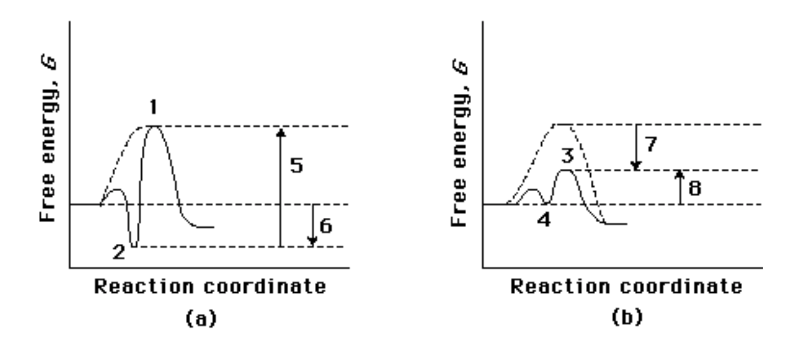Compare the two reaction coordinate diagrams below and select the answer that correctly describes their relationship. In each case, the single intermediate is the ES complex.

(a) describes a strict “lock and key” model, whereas (b) describes a transition-state complementarity model.

24

Which of the following is true of the binding energy derived from enzyme-substrate interactions?

It is sometimes used to hold two substrates in the optimal orientation for reaction.

25

The concept of “induced fit” refers to the fact that:

substrate binding may induce a conformational change in the enzyme, which then brings catalytic groups into proper orientation.

26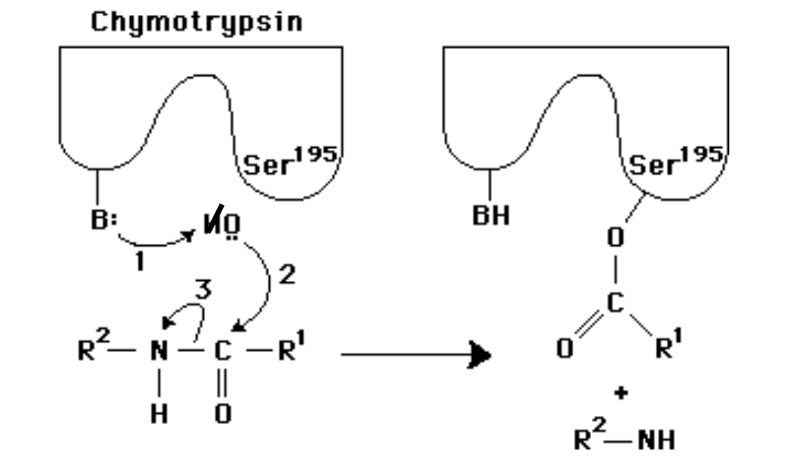In the following diagram of the first step in the reaction catalyzed by the protease chymotrypsin, the process of general base catalysis is illustrated by the number ________, and the process of covalent catalysis is illustrated by the number _________.

1;2

27

The benefit of measuring the initial rate of a reaction V0 is that at the beginning of a reaction:

changes in [S] are negligible, so [S] can be treated as a constant.

28

Which of the following statements about a plot of V0 vs. [S] for an enzyme that follows Michaelis- Menten kinetics is false?

At very high [S], the velocity curve becomes a horizontal line that intersects the y-axis at Km.

29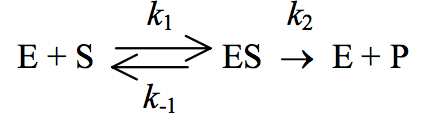Michaelis and Menten assumed that the overall reaction for an enzyme-catalyzed reaction could be written as

Using this reaction, the rate of breakdown of the enzyme-substrate complex can be described by the expression:

k-1 [ES] + k2 [ES].

30

The steady state assumption, as applied to enzyme kinetics, implies:

the ES complex is formed and broken down at equivalent rates.

31

An enzyme-catalyzed reaction was carried out with the substrate concentration initially a thousand times greater than the Km for that substrate. After 9 minutes, 1% of the substrate had been converted to product, and the amount of product formed in the reaction mixture was 12 μmol. If, in a separate experiment, one-third as much enzyme and twice as much substrate had been combined, how long would it take for the same amount (12 μmol) of product to be formed?

27 min

32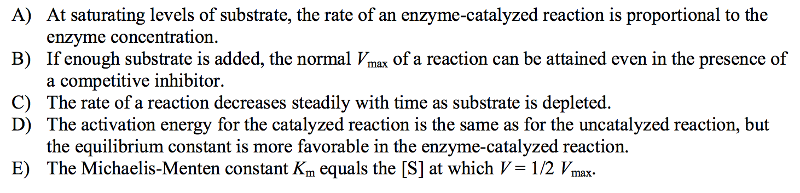Which of these statements about enzyme-catalyzed reactions is false?

The activation energy for the catalyzed reaction is the same as for the uncatalyzed reaction, but the equilibrium constant is more favorable in the enzyme-catalyzed reaction.

33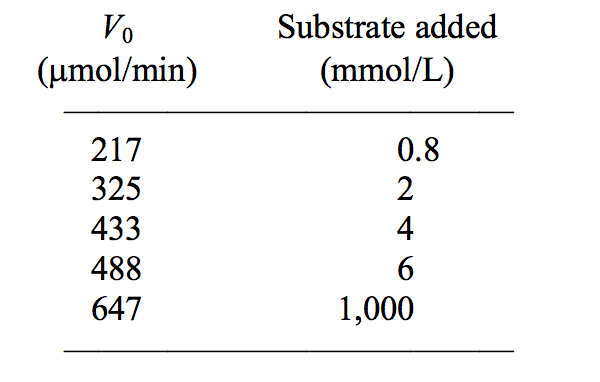The following data were obtained in a study of an enzyme known to follow Michaelis-Menten kinetics:

The Km for this enzyme is approximately:

2mM.

34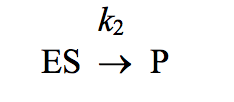For enzymes in which the slowest (rate-limiting) step is the reaction

Km becomes equivalent to:

the dissociation constant, Kd, for the ES complex.

35

The Lineweaver-Burk plot is used to:

solve, graphically, for the rate of an enzymatic reaction at infinite substrate concentration.

36The double-reciprocal transformation of the Michaelis-Menten equation, also called the Lineweaver- Burk plot, is given by

multiply the reciprocal of the x-axis intercept by −1.

37

To calculate the turnover number of an enzyme, you need to know:

- the enzyme concentration.

- the initial velocity of the catalyzed reaction at [S] >> Km.

38

The number of substrate molecules converted to product in a given unit of time by a single enzyme molecule at saturation is referred to as the:

turnover number.

39

In a plot of l/V against 1/[S] for an enzyme-catalyzed reaction, the presence of a competitive inhibitor will alter the:

intercept on the l/[S] axis.

40

In competitive inhibition, an inhibitor:

binds reversibly at the active site.

41

Vmax for an enzyme-catalyzed reaction:

is twice the rate observed when the concentration of substrate is equal to the Km.

42

Enzyme X exhibits maximum activity at pH = 6.9. X shows a fairly sharp decrease in its activity when the pH goes much lower than 6.4. One likely interpretation of this pH activity is that:

a His residue on the enzyme is involved in the reaction.

43

Both water and glucose share an —OH that can serve as a substrate for a reaction with the terminal phosphate of ATP catalyzed by hexokinase. Glucose, however, is about a million times more reactive as a substrate than water. The best explanation is that:

the larger glucose binds better to the enzyme; it induces a conformational change in hexokinase

that brings active-site amino acids into position for catalysis.

44

A good transition-state analog:

binds to the enzyme more tightly than the substrate.

45

A transition-state analog:

resembles the transition-state structure of the normal enzyme-substrate complex.

46

The role of the metal ion (Mg2+) in catalysis by enolase is to

facilitate general base catalysis

47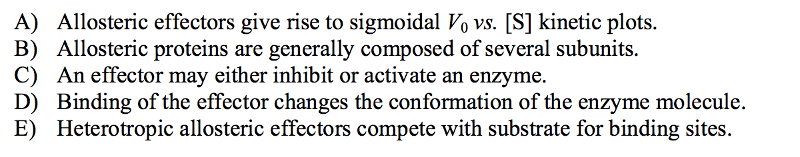Which of the following statements about allosteric control of enzymatic activity is false?

Heterotropic allosteric effectors compete with substrate for binding sites.

48

A small molecule that decreases the activity of an enzyme by binding to a site other than the catalytic site is termed a(n):

allosteric inhibitor.

49

Allosteric enzymes:

usually have more than one polypeptide chain.

50

A metabolic pathway proceeds according to the scheme, R → S → T → U → V → W. A regulatory enzyme, X, catalyzes the first reaction in the pathway. Which of the following is most likely correct for this pathway?

The last product, W, is likely to be a negative modulator of X, leading to feedback inhibition.

51

Which of the following has not been shown to play a role in determining the specificity of protein kinases?

Disulfide bonds near the phosphorylation site

52

How is trypsinogen converted to trypsin?

Proteolysis of trypsinogen forms trypsin.

53

Define the terms “cofactor” and “coenzyme.”

A cofactor is any chemical component required for enzyme activity; it includes both organic molecules, called “coenzymes,” and inorganic ions.

54

Draw and label a reaction coordinate diagram for an uncatalyzed reaction, S → P, and the same reaction catalyzed by an enzyme, E.

...

55

The difference in (standard) free energy content, ∆G'°, between substrate S and product P may vary considerably among different reactions. What is the significance of these differences?

The difference in free energy content between substrate (or reactant) and product for each reaction reflects the relative amounts of each compound present at equilibrium. The greater the difference in free energy, the greater the difference in amounts of each compound at equilibrium.

56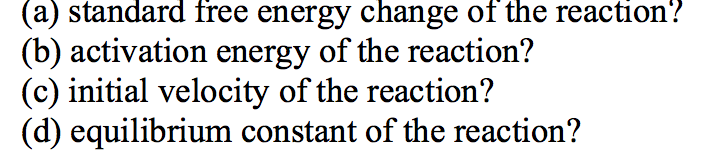For a reaction that can take place with or without catalysis by an enzyme, what would be the effect of the enzyme on the:

(a) no change; (b) decrease; (c) increase; (d) no change

57

Sometimes the difference in (standard) free-energy content, ∆G'°, between a substrate S and a product P is very large, yet the rate of chemical conversion, S → P, is quite slow. Why?

the rate of conversion from substrate to product (or the reverse reaction, from product to substrate) does not depend on the free-energy difference between them. The rate of the reaction

depends upon the activation energy of the reaction ∆G'‡, which is the difference between the free- energy content of S (or P) and the reaction transition state.

58

Write an equilibrium expression for the reaction S → P and briefly explain the relationship between the value of the equilibrium constant and free energy.

keq' = [P]/[S]. The value of Keq' reflects the difference between the free energy content of S and P. Free energy and equilibrium constant are related by the expression:

∆G'° = -RT ln Keq'
For each change in Keq' by one order of magnitude, ∆G'° changes by 5.7 Kjoule/mole.

59

What is the difference between general acid-base catalysis and specific acid-base catalysis? (Assume that the solvent is water.)

Specific acid-base catalysis refers to catalysis by the constituents of water, i.e., the donation of a proton by the hydronium ion, H3O+ or the acceptance of a proton by the hydroxyl ion OH-. General acid- base catalysis refers to the donation or acceptance of a proton by weak acids and bases other than water.

60

Michaelis-Menten kinetics is sometimes referred to as “saturation” kinetics. Why?

According to the Michaelis-Menten model of enzyme-substrate interaction, when [S] becomes very high, an enzyme molecule's active site will become occupied with a new substrate molecule as

soon as it releases a product. Therefore, at very high [S], V0 does not increase with additional

substrate, and the enzyme is said to be “saturated” with substrate.

61

Two different enzymes are able to catalyze the same reaction, A → B. They both have the same Vmax, but differ their Km the substrate A. For enzyme 1, the Km is 1.0 mM; for enzyme 2, the Km is 10 mM. When enzyme 1 was incubated with 0.1 mM A, it was observed that B was produced at a rate of 0.0020 mmoles/minute. a) What is the value of the Vmax of the enzymes? b) What will be the rate of production of B when enzyme 2 is incubated with 0.1 mM A? c) What will be the rate of production of B when enzyme 1 is incubated with 1 M (i.e., 1000 mM) A?

a) 0.022 mmol/min; b) 0.0022 mmol/min; c) 0.022 mmol/min

62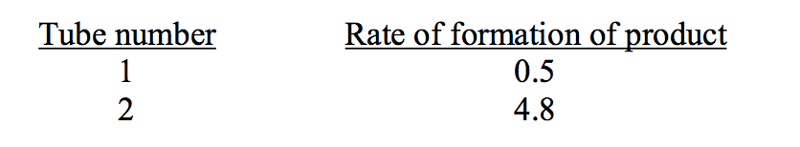An enzyme can catalyze a reaction with either of two substrates, S1 or S2. The Km for S1 was found to be 2.0 mM, and the Km, for S2 was found to be 20 mM. A student determined that the Vmax was the same for the two substrates. Unfortunately, he lost the page of his notebook and needed to know the value of Vmax. He carried out two reactions: one with 0.1 mM S1, the other with 0.1 mM S2. Unfortunately, he forgot to label which reaction tube contained which substrate. Determine the value of Vmax from the results he obtained:

Vmax = 101

63

Write out the equation that describes the mechanism for enzyme action used as a model by Michaelis and Menten. List the important assumptions used by Michaelis and Menten to derive a rate equation for this reaction.

The two equations are k1 k2

E+ ES E+P k-1 k-2

One assumption is that [P] = 0, so that the rate of the reaction depends exclusively on the breakdown of ES and is not influenced by the reverse reaction; that is, k-2 can be ignored and V0 = k2 [ES]. This condition is possible only if early reaction times are measured; the velocity, therefore, is an initial velocity. A second assumption is that the rate of ES formation equals the rate of ES breakdown; in other words, the reaction is at a steady state. A third assumption is [S] >> [Et], so that total [S], which equals free substrate and enzyme-bound substrate, is essentially equal to [S].

64

Give the Michaelis-Menten equation and define each term in it. Does this equation apply to all enzymes? If not, to which kind does it not apply?

The Michaelis-Menten equation is: V0 = Vmax [S]/( Km + [S]), in which V0 is the initial velocity at any given concentration of S, Vmax is the velocity when all enzyme molecules are saturated with S, [S] is the concentration of S, and Km is a constant characteristic for the enzyme. This equation does not apply to enzymes that display sigmoidal V0 vs. [S] curves, but only to those giving hyperbolic kinetic plots.

65

An enzyme catalyzes a reaction at a velocity of 20 μmol/min when the concentration of substrate (S) is 0.01 M. The Km for this substrate is 1 × 10-5 M. Assuming that Michaelis-Menten kinetics are followed, what will the reaction velocity be when the concentration of S is (a) 1 × 10-5 M and (b) 1 × 10-6 M?

The velocity of 20 μmol/min is essentially Vmax because it is measured at [S] >> Km. (a) When [S] = 10-5 M = Km, V = 1/2 Vmax, or 10 μmol/min. (b) When [S] is 10-6 M, velocity can be calculated from the Michaelis-Menten equation:

V0 = Vmax [S]/( Km + [S]) = (20 μmol/min)(10-6 M)/(10-5 + 10-6) = 1.8 μmol/min.

66

For the reaction E + S → ES → P the Michaelis-Menten constant, Km, is actually a summary of three terms. What are they? How is Km determined graphically?

Km = (k2 + k-1)/ k1, where k-1 and k1 are the rate constants for the breakdown and association,

respectively, of the ES complex and k2 is the rate constant for the breakdown of ES to form E + P. Km can be determined graphically on a plot of V0 vs. [S] by finding the [S] at which V0 = 1/2 Vmax. More conveniently, on a double-reciprocal plot, the x-axis intercept = –1/ Km.

67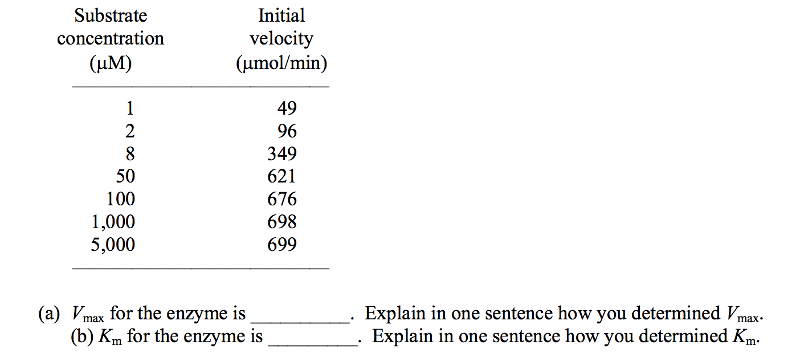A biochemist obtains the following set of data for an enzyme that is known to follow Michaelis- Menten kinetics.

(a) Vmax is about 700. In a plot of V vs. [S], the asymptote is Vmax. Simple inspection of the datashows the approach to Vmax—the rate increases by only 1 unit when [S] increases fivefold.

(b) Km is about 8 μM, the [S] at which the velocity is half-maximal. Because Vmax is about 700,

1/2 Vmax is about 350. The [S] at that rate is about 8 μM.

68

Why is the Lineweaver-Burk (double reciprocal) plot (see Box 6, p. 206) more useful than the standard V vs. [S] plot in determining kinetic constants for an enzyme? (Your answer should probably show typical plots.)

The plot of V vs. [S] is hyperbolic; maximum velocity is never achieved experimentally, because it is impossible to do experiments at infinitely high [S]. The Lineweaver-Burk transformation of the Michaelis-Menten equation produces a linear plot that can be extrapolated to infinite [S] (where 1/[S] becomes zero), allowing a determination of Vmax.

69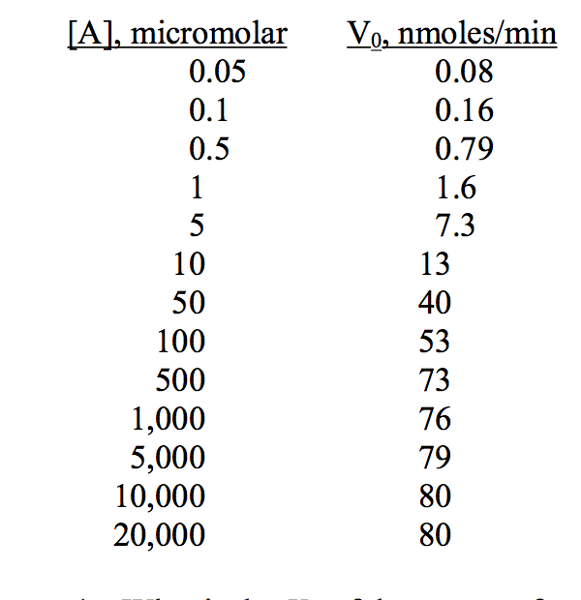An enzyme catalyzes the reaction A → B. The initial rate of the reaction was measured as a function of the concentration of A. The following data were obtained:

a)

What is the Km of the enzyme for the substrate A?

b)
The above data was plotted as 1/ V0 vs. 1/[A], and a straight line was obtained.

What is the value of V0 when [A] = 43?
c) What is the value of the y-intercept of the line?

d) What is the value of the x-intercept of the line?

a) 50 micromolar; b) 37 nmoles/min; c) 0.0125 (nmole/min)-1; d) −0.02 micromolar-1

70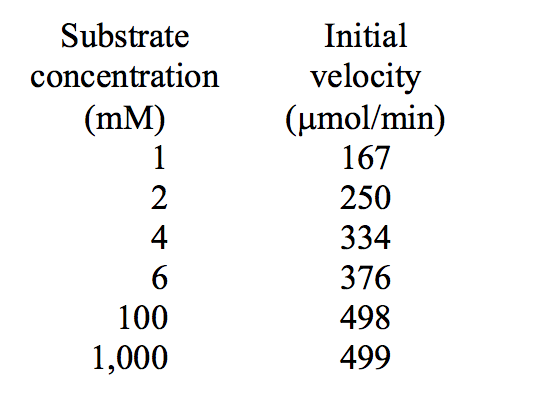The turnover number for an enzyme is known to be 5,000 min-1. From the following set of data, calculate the Km and the total amount of enzyme present in these experiments.

(a) Km = __________. (b) Total enzyme = __________ μmol.

Km = about 2 mM (the concentration of S needed to achieve one-half of Vmax, which is about 500). The total enzyme present is producing about 500 μmol of product per minute. Because the turnover number is 5,000/min, the amount of enzyme present must be 0.1 μmol; 1 μmol of enzyme would produce 5,000 μmol product/min.

71

When 10 μg of an enzyme of Mr 50,000 is added to a solution containing its substrate at a concentration one hundred times the Km, it catalyzes the conversion of 75 μmol of substrate into product in 3 min. What is the enzyme's turnover number?

Because the velocity measured occurs far above Km, it represents Vmax. Ten μg of the enzyme represents 10 × 10-6 g/(5 × 104 g/mol), or 2 × 10-10 mol of enzyme. In 3 minutes, this amount of enzyme produced 75 μmol of product, equivalent to 25 × 10-6 mol of product per minute. The turnover number is therefore

(25 × 10-6 mol/min)/(2 × 10-10 mol) = 12.5 × 104 min-1.

72

Fifteen μg of an enzyme of Mr 30,000 working at Vmax catalyzes the conversion of 60 μmol of substrate into product in 3 min. What is the enzyme's turnover number?

The amount of enzyme present is 15 × 10-6 g, which is (15 × 10-6 g)/(3 × 104 g/mol) = 5 × 10-10 mol of enzyme. The rate of product formation is 60 × 10-6 mol/3 min, or 20 × 10-6 mol of product per minute. The turnover number is therefore (20 × 10-6 mol/min)/(5 × 10-10 mol of enzyme), or 4 × 10-4 min-1.

73

How does the total enzyme concentration affect turnover number and Vmax?

The turnover number, kcat, is the number of substrate molecules converted to product in a given time by a single enzyme molecule, so turnover number is not affected by the total enzyme concentration, [Et]. For any given reaction, however, Vmax can change because Vmax is the product of turnover number × the total enzyme concentration, or Vmax = kcat [Et].

74

Enzymes with a kcat / Km ratio of about 108 M-1s-1 are considered to show optimal catalytic efficiency. Fumarase, which catalyzes the reversible-dehydration reaction

fumarate + H2O malate
has a ratio of turnover number to the Michaelis-Menten constant, (kcat / Km) of 1.6 × 108 for the substrate fumarate and 3.6 × 107 for the substrate malate. Because the turnover number for both substrates is nearly identical, what factors might be involved that explain the different ratio for the two substrates?

If the turnover number is nearly identical for both substrates, then the Km for malate must be much larger than for fumarate. Similar turnover numbers suggest no significant differences in rate of conversion of substrate to product, but the different Km values could possibly be explained by a stronger binding affinity of the enzyme for fumarate than for malate or some other aspect of the reaction mechanism that affects Km.

75

Methanol (wood alcohol) is highly toxic because it is converted to formaldehyde in a reaction catalyzed by the enzyme alcohol dehydrogenase:

Part of the medical treatment for methanol poisoning is to administer ethanol (ethyl alcohol) in amounts large enough to cause intoxication under normal circumstances. Explain this in terms of what you know about examples of enzymatic reactions.

Ethanol is a structural analog of methanol, and competes with methanol for the binding site of alcohol dehydrogenase, slowing the conversion of methanol to formaldehyde, and allowing its clearance by the kidneys. The effect of ethanol is that of a competitive inhibitor.

76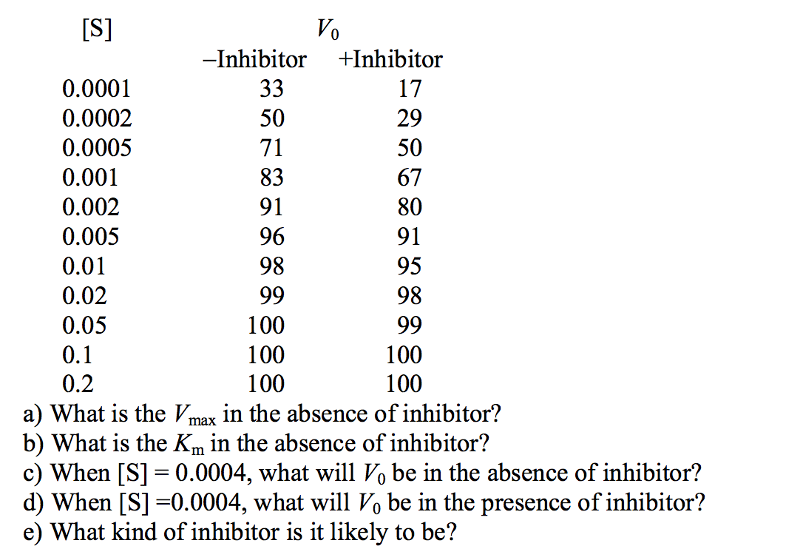You measure the initial rate of an enzyme reaction as a function of substrate concentration in the presence and absence of an inhibitor. The following data are obtained:

a) 100; b) 0.0002; c) 66.7; d) 40; e) competitive

77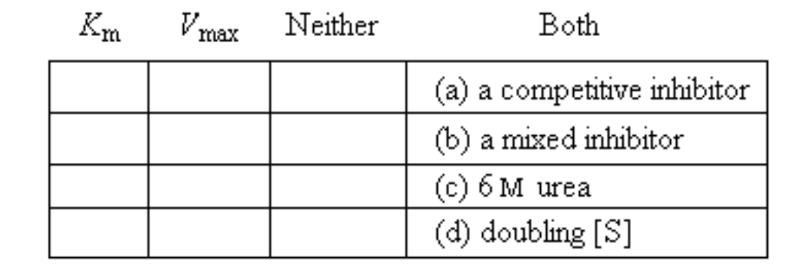An enzyme follows Michaelis-Menten kinetics. Indicate (with an "x") which of the kinetic parameters at the left would be altered by the following factors. Give only one answer for each.

(a) Km; (b) both; (c) both; (d) neither

78

The enzymatic activity of lysozyme is optimal at pH 5.2 and decreases above and below this pH value. Lysozyme contains two amino acid residues in the active site essential for catalysis: Glu35 and Asp52. The pK value for the carboxyl side chains of these two residues are 5.9 and 4.5, respectively. What is the ionization state of each residue at the pH optimum of lysozyme? How can the ionization states of these two amino acid residues explain the pH-activity profile of lysozyme?

For the enzyme to be active, it is likely that Asp52 is unprotonated and Glu35 is protonated. When the pH is below 4.5, Asp52 becomes protonated, and when it is above 5.9, Glu35 is deprotonated, either of which decreases the activity of the enzyme. (

79

Why does pH affect the activity of an enzyme?

The state of ionization of several amino acid side chains is affected by pH, and the activity of many enzymes requires that certain of the amino acid residue side chains be in a specific ionization state

80

Chymotrypsin belongs to a group of proteolytic enzymes called the “serine proteases,” many of which have an Asp, His, and Ser residue that are crucial to the catalytic mechanism. The serine hydroxyl functions as a nucleophile. What do the other two amino acids do to support this nucleophilic reaction?

In chymotrypsin, histidine functions as a general base, accepting a proton from the serine hydroxyl, thereby increasing serine's reactivity as a nucleophile. The negatively charged Asp stabilizes the positive charge that develops on the His.

81

For serine to work effectively as a nucleophile in covalent catalysis in chymotrypsin a nearby amino acid, histidine, must serve as general base catalyst. Briefly describe, in words, how these two amino acids work together.

The serine is a polar hydroxyl, with the oxygen functioning as an electronegative nucleophile. A nearby histidine residue, with pKa ≈ 6.0, however, functions as a base to abstract the proton from the serine hydroxyl group. The result is to substantially increase the electronegativity of the serine oxygen, making it a much stronger nucleophile. This, in turn, lowers the activation energy of the covalent catalysis between serine and the carbonyl carbon of the substrate peptide bond

82

On the enzyme hexokinase, ATP reacts with glucose to produce glucose 6-phosphate and ADP five orders of magnitude faster than ATP reacts with H2O to form phosphate and ADP. The intrinsic chemical reactivity of the —OH group in water is about the same as that of the glucose molecule, and water can certainly fit into the active site. Explain this rate differential in two sentences or less.

The binding of glucose to hexokinase induces a conformation change that brings the amino acid residues that facilitate the phosphoryl transfer into position in the active site. Binding of water alone does not induce this conformational change.

83

Why is a transition-state analog not necessarily the same as a competitive inhibitor?

The structure of a competitive inhibitor may be similar to the structure of the free substrate. Similar structure will mean that the competitive inhibitor can associate with the enzyme at the active site, effectively blocking the normal substrate from binding. A transition-state analog, however, is similar in structure to the transition-state of the reaction catalyzed by the enzyme. Often a transition- state analog will bind tightly to an enzyme, and is not easily competed away by substrate.

84

The scheme S → T → U → V → W → X → Y represents a hypothetical pathway for the metabolic synthesis of compound Y. The pathway is regulated by feedback inhibition. Indicate where the inhibition is most likely to occur and what the likely inhibitor is.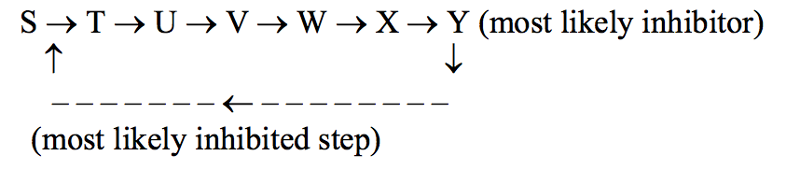85

Explain how a biochemist might discover that a certain enzyme is allosterically regulated.

The enzyme would show kinetics that do not fit the Michaelis-Menten equation; the plot of V vs. [S] would be sigmoidal, not hyperbolic. The enzyme kinetics would be affected by molecules other than the substrate(s).

86

What is a zymogen (proenzyme)? Explain briefly with an example.

A zymogen is an inactive form of an enzyme that is activated by one or more proteolytic cleavages in its sequence. Chymotrypsinogen, trypsinogen, and proelastase are all zymogens, becoming chymotrypsin, trypsin, and elastase, respectively, after proper cleavage.